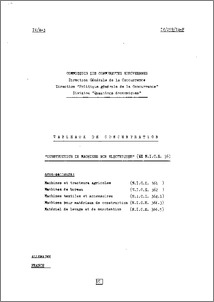# Construction de machines non electriques (Ex N.I.C.E. 36). Machines et tracteurs agricoles (N.I.C.E. 36). Machines de bureau (N.I.C.E. 362). Machines textiles et accessoires (N.I.C.E. 364.1). Machines pour materiaux de construction (N.I.C.E. 366.3). Material de levage et de manutention (N.I.C.E. 366.5). Tableaux de concentration. Allemagne. France = Construction of non-electrical machinery (Ex N.I.C.E. 36). Agricultural machinery and tractors (N.I.C.E. 36). Office machines (N.I.C.E. 362). Textile machinery and accessories (N.I.C.E. 364.1). Construction machinery (N.I.C.E. 366.3). Lifting and material handling (N.I.C.E. 366.5). Concentration tables. Germany. France. Studies: Evolution of concentration and competition series

UNSPECIFIED (1974) Construction de machines non electriques (Ex N.I.C.E. 36). Machines et tracteurs agricoles (N.I.C.E. 36). Machines de bureau (N.I.C.E. 362). Machines textiles et accessoires (N.I.C.E. 364.1). Machines pour materiaux de construction (N.I.C.E. 366.3). Material de levage et de manutention (N.I.C.E. 366.5). Tableaux de concentration. Allemagne. France = Construction of non-electrical machinery (Ex N.I.C.E. 36). Agricultural machinery and tractors (N.I.C.E. 36). Office machines (N.I.C.E. 362). Textile machinery and accessories (N.I.C.E. 364.1). Construction machinery (N.I.C.E. 366.3). Lifting and material handling (N.I.C.E. 366.5). Concentration tables. Germany. France. Studies: Evolution of concentration and competition series. [EU Commission - Working Document]Preview
PDF - Published Version